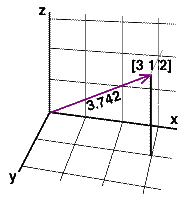VectorsNormalizing

Vector Length

Figure 1 shows a fixed vector with the following coordinates ie. components,
a[3 1 2]
in other words,
ax = 3,
ay = 1,
az = 2,Figure 1

The magnitude (length) of the vector is,

length = sqrt((ax * ax) + (ay * ay) + (az * az))
length = sqrt(9 + 1 + 4) = 3.742

As a short-hand notation the magnitude of a vector is written with two vertical lines,

|a| = sqrt((ax * ax) + (ay * ay) + (az * az))

Unit Vectors - Normalizing

Operations in 2D and 3D computer graphics are often performed using copies of vectors that have been normalized ie. converted to unit vectors. For example, the tutorial "RSL: Edge Effects" applies normalization before calculating the dot product of two vectors. Normalizing a vector involves two steps:
1   calculate its length, then,
2   divide each of its (xy or xyz) components by its length.

Given vector a its xyz components are calculated as follows,

x = ax/|a|
y = ay/|a|
z = az/|a|

As a "worked example" the vector shown in figure 1 has the xyz components of 3, 1, 2 and a length of 3.742. Therefore, a normalized copy of the vector will have components,

x = 3.0 / 3.742 = 0.802
y = 1.0 / 3.742 = 0.267
z = 2.0 / 3.742 = 0.534